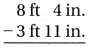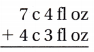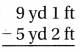Refer to our Texas Go Math Grade 4 Answer Key Pdf to score good marks in the exams. Test yourself by practicing the problems from Texas Go Math Grade 4 Module 15 Assessment Answer Key.

Vocabulary

• milliliter
• millimeter
• pint
• pound
• yard

Choose the best term from the box to complete the sentence.

Question 1.
A ______________ is a customary unit used to measure weight.
Answer: the ounce (oz) and pound (lb).
Explanation:
A ounce (oz) and pound (lb) is a customary unit used to measure weight.

Question 2.
The cup and the ______________ are both customary units for measuring liquid volume.
Explanation:
The cup and the Pint are both customary units for measuring liquid volume.

Question 3.
A ______________ is a metric unit for measuring length or distance.
Explanation:
A meter is a metric unit for measuring length or distance.

Question 4.
A ______________ is a metric unit for measuring liquid volume. p. 555)
Explanation:
A liter is a metric unit for measuring liquid volume

Concepts and Skills

Circle the better estimate.

Question 5.
weight of a school bus 11 tons or 1 ton
Explanation:
weight of a school bus is approx. 11 ton

Question 6.
distance between EI Paso and Fort Worth 60 miles or 605 miles
Explanation:
Distance between EI Paso and Fort Worth is 605 miles

Question 7.
height of your desk 1 meter or 3 meters
Explanation:
height of my desk is 1 meter

Question 8.
the amount of liquid a coffee cup holds 800 fluid ounces or 8 fluid ounces
Explanation:
the amount of liquid a coffee cup holds  8 fluid ounces

Complete the sentence. Write more or less.

Question 9.
A cat weighs ___________ than one ounce.
Explanation:
A cat weighs more than one ounce.

Question 10.
Serena’s shoe is ___________ one yard long.
Explanation:
Serena’s shoe is less than one yard long.
Complete.

Question 11.
5 feet = ___________ inches
Explanation:
1 feet = 12 inches
5 feet = 5 x 12 inches = 60 inches

Question 12.
4 tons = ___________ pounds
Explanation:
1 ton = 2000 Pounds
4 tons = 4 x 2000 =  8000 pounds
Question 13.
4 cups = ___________ pints
Explanation:
1 cup = 0.5 pint
4 cups = 4 x 0.5 =2 pints
Question 14.
4,000 pounds = ___________ tons
Explanation:
1 ton = 2000 Pounds
4,000 pounds = 4000 ÷2000 = 2 tons

Question 15.
2 quarts = ___________ cups
Explanation:
1 quart = 4 cups
2 quarts = 2 x 4 =8 cups

Question 16.
36 inches = ___________ feet
Explanation:
12 inches = 1 feet
36 inches = 36 ÷ 12 =  3 feet

Question 17.
3 meters = ___________ decimeters
Explanation:
1 meter = 10 decimeters
3 meters = 30 decimeters

Question 18.
40 centimeters = ___________ decimeters
Explanation:
1 centimeter = 10 decimeters
40 centimeters = 4 decimeters

Question 19.
7 kilograms = ___________ grams
Explanation:
1 kilogram = 1000 grams
7 kilograms = 7 x 1000 = 7000 grams

Question 20.Explanation:
8 ft 4 in= 7 ft 16 in
so 7 ft 16 in – 3ft 11 in= 4ft 5 in

Question 21.Answer: 11 C 7 fl oz
Explanation:
7 C 4 fl oz + 4 C 3 fl oz = 11 C 7 fl oz

Question 22.Explanation:
1 yd = 3 ft
9 yd 1ft = 8 yd 4ft
8 yd 4ft – 5 yd 2 ft =3 yd 2 ft

Question 23.
A jug contains 8 quarts of water. How many gallons of water does the jug contain?
(A) 4 gallons
(B) 2 gallons
(C) 3 gallons
(D) 1 gallon
Explanation:
1 gallon = 4 quarts
8 quarts = 8 ÷4 = 2 gallons
Question 24.
Serena bought 4 pounds of dough to make pizzas. The recipe gives the amount of dough needed for a pizza in ounces. How many ounces of dough did she buy?
(A) 8 ounces
(B) 96 ounces
(C) 64 ounces
(D) 16 ounces
Explanation:
1 pound = 16 ounces
4 pounds = 4 x16= 96  ounces

Question 25.
Kainoa bought a brick of modeling clay that was labeled 2 kilograms. He needs to separate the clay into balls that are measured in grams. How many grams does he have?
(A) 20,000 grams
(B) 200 grams
(C) 2,000 grams
(D) 20 grams
Explanation:
1 kilogram = 1000 grams
2 kilograms = 2000 grams

Question 26.
Lewis fills his thermos with 2 liters of water. Garret fills his thermos with 1 liter of water. How many more milliliters of water does Lewis have than Garret?
(A) 1 more milliliter
(B) 2,000 more milliliters
(C) 1,000 more milliliters
(D) 100 more milliliters